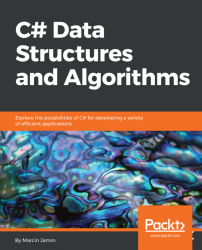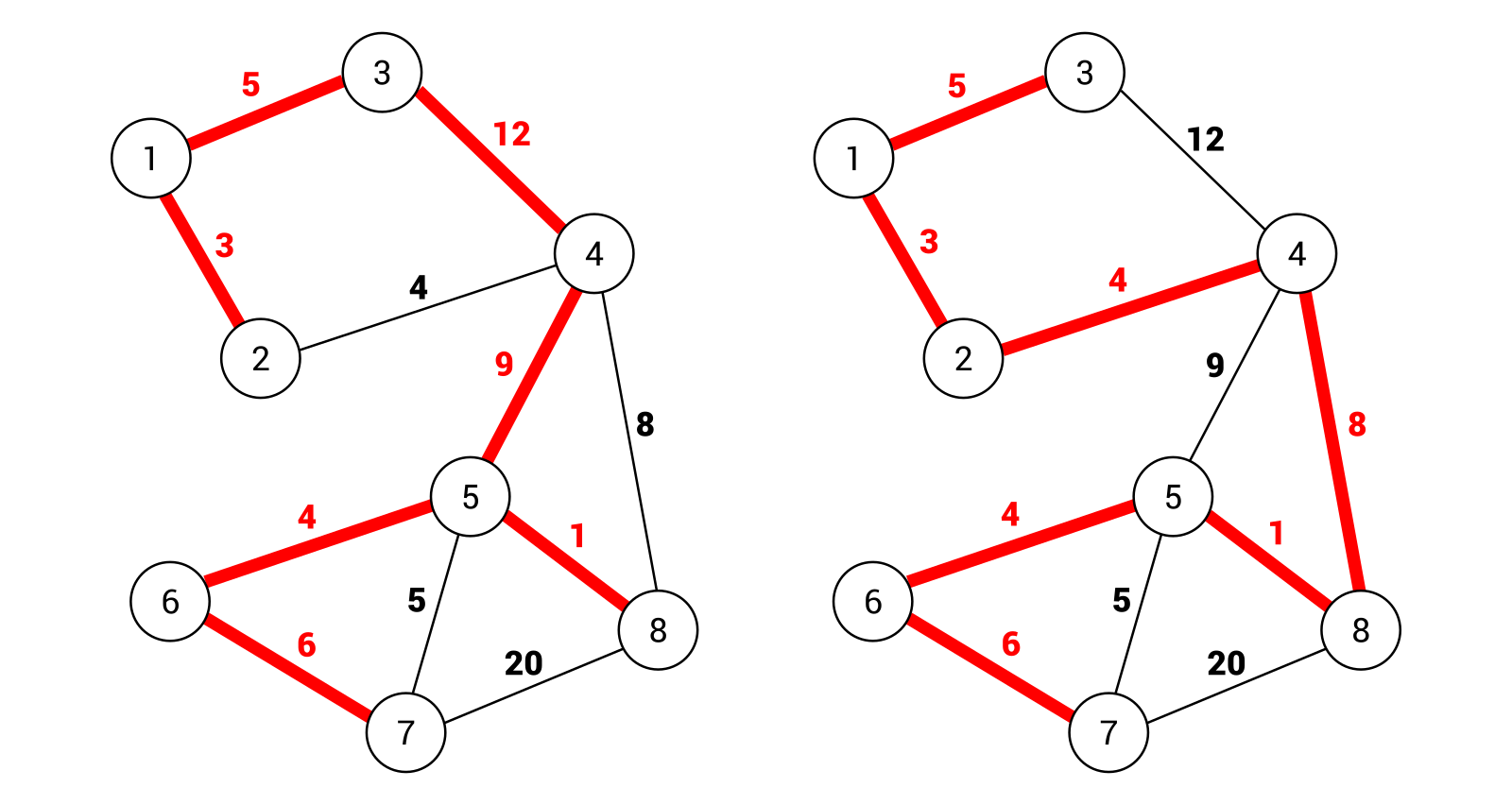•#### C# Data Structures and Algorithms#### Overview of this book

Data structures allow organizing data efficiently. They are critical to various problems and their suitable implementation can provide a complete solution that acts like reusable code. In this book, you will learn how to use various data structures while developing in the C# language as well as how to implement some of the most common algorithms used with such data structures. At the beginning, you will get to know arrays, lists, dictionaries, and sets together with real-world examples of your application. Then, you will learn how to create and use stacks and queues. In the following part of the book, the more complex data structures will be introduced, namely trees and graphs, together with some algorithms for searching the shortest path in a graph. We will also discuss how to organize the code in a manageable, consistent, and extendable way. By the end of the book,you will learn how to build components that are easy to understand, debug, and use in different applications.
Title PagePackt UpsellContributorsPrefaceFree Chapter
Getting StartedArrays and ListsStacks and QueuesDictionaries and SetsVariants of TreesExploring GraphsSummaryOther Books You May EnjoyIndex## Minimum spanning tree

While talking about graphs, it is beneficial to introduce the subject of a spanning tree. What is it? A spanning tree is a subset of edges that connects all nodes in a graph without cycles. Of course, it is possible to have many spanning trees within the same graph. For example, let's take a look at the following diagram:On the left-hand side is a spanning tree that consists of the following edges: (1, 2), (1, 3), (3, 4), (4, 5), (5, 6), (6, 7), and (5, 8). The total weight is equal to 40. On the right-hand side, another spanning tree is shown. Here, the following edges are taken into account: (1, 2), (1, 3), (2, 4), (4, 8), (5, 8), (5, 6), and (6, 7). The total weight is equal to 31.

However, neither of the preceding spanning trees is the minimum spanning tree (MST) of this graph. What does it mean that a spanning tree is minimum? The answer is really simple: it is a spanning tree with the minimum cost from all spanning trees available in the graph. You can get the...